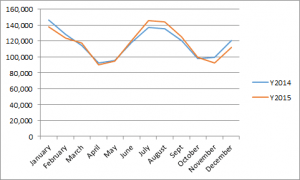26
Dec 16

## Multiple regression through the prism of dummy variables

Agresti and Franklin on p.658 say: The indicator variable for a particular category is binary. It equals 1 if the observation falls into that category and it equals 0 otherwise. I say: For most students, this is not clear enough.

### Problem statementFigure 1. Residential power consumption in 2014 and 2015. Source: http://www.eia.gov/electricity/data.cfm

Residential power consumption in the US has a seasonal pattern. Heating in winter and cooling in summer cause the differences. We want to capture the dependence of residential power consumption$PowerC$ on the season.

### Visual approach to dummy variables

Seasons of the year are categorical variables. We have to replace them with quantitative variables, to be able to use in any mathematical procedure that involves arithmetic operations. To this end, we define a dummy variable (indicator)$D_{win}$ for winter such that it equals 1 in winter and 0 in any other period of the year. The dummies$D_{spr},\ D_{sum},\ D_{aut}$ for spring, summer and autumn are defined similarly. We provide two visualizations assuming monthly observations.

 Month$D_{win}$$D_{win}$$D_{spr}$$D_{spr}$$D_{sum}$$D_{sum}$$D_{aut}$$D_{aut}$$D_{win}+D_{spr}+ D_{sum}+D_{aut}$$D_{win}+D_{spr}+ D_{sum}+D_{aut}$ December 1 0 0 0 1 January 1 0 0 0 1 February 1 0 0 0 1 March 0 1 0 0 1 April 0 1 0 0 1 May 0 1 0 0 1 June 0 0 1 0 1 July 0 0 1 0 1 August 0 0 1 0 1 September 0 0 0 1 1 October 0 0 0 1 1 November 0 0 0 1 1Figure 2. Graphical visualization of D_spr

### The first idea may be wrong

The first thing that comes to mind is to regress$PowerC$ on dummies as in

(1)$PowerC=a+bD_{win}+cD_{spr}+dD_{sum}+eD_{aut}+error.$

Not so fast. To see the problem, let us rewrite (1) as

(2)$PowerC=a\times 1+bD_{win}+cD_{spr}+dD_{sum}+eD_{aut}+error.$

This shows that, in addition to the four dummies, there is a fifth variable, which equals 1 across all observations. Let us denote it$T$ (for Trivial). Table 1 shows that

(3)$T=D_{win}+D_{spr}+ D_{sum}+D_{aut}.$

This makes the next definition relevant. Regressors$x_1,...,x_k$ are called linearly dependent if one of them, say,$x_1$, can be expressed as a linear combination of the others:$x_1=a_2x_2+...+a_kx_k$.  In case (3), all coefficients$a_i$ are unities, so we have linear dependence. Using (3), let us replace$T$ in (2). The resulting equation is rearranged as

(4)$PowerC=(a+b)D_{win}+(a+c)D_{spr}+(a+d)D_{sum}+(a+e)D_{aut}+error.$

Now we see what the problem is. When regressors are linearly dependent, the model is not uniquely specified. (1) and (4) are two different representations of the same model.

### What is the way out?

If regressors are linearly dependent, drop them one after another until you get linearly independent ones. For example, dropping the winter dummy, we get

(5)$PowerC=a+cD_{spr}+dD_{sum}+eD_{aut}+error.$

Here is the estimation result for the two-year data in Figure 1:$PowerC=128176-27380D_{spr}+5450D_{sum}-22225D_{aut}$.

This means that:$PowerC=128176$ in winter,$PowerC=128176-27380$ in spring,$PowerC=128176+5450$ in summer, and$PowerC=128176-22225$ in autumn.

It is revealing that cooling requires more power than heating. However, the summer coefficient is not significant. Here is the Excel file with the data and estimation result.

The category that has been dropped is called a base (or reference) category. Thus, the intercept in (5) measures power consumption in winter. The dummy coefficients in (5) measure deviations of power consumption in respective seasons from that in winter.

### Here is the question I ask my students

We want to see how beer consumption$BeerC$ depends on gender and income$Inc$. Let$M$ and$F$ denote the dummies for males and females, resp. Correct the following model and interpret the resulting coefficients:$BeerC=a+bM+cF+dM^2+eF^2+fFM+(h+iM)Inc.$

### Final remark

When a researcher includes all categories plus the trivial regressor, he/she falls into what is called a dummy trap. The problem of linear dependence among regressors is usually discussed under the heading of multiple regression. But since the trivial regressor is present in simple regression too, it might be a good idea to discuss it earlier.

Linear dependence/independence of regressors is an exact condition for existence of the OLS estimator. That is, if regressors are linearly dependent, then the OLS estimator doesn't exist, in which case the question about its further properties doesn't make sense. If, on the other hand, regressors are linearly independent, then the OLS estimator exists, and further properties can be studied, such as unbiasedness, variance and efficiency.